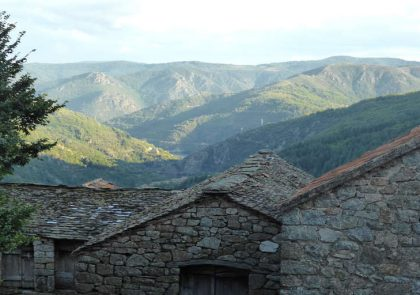# Blog

We provide practical tutorials on data mining, visualization and statistics for decision making.This article describes how to remove legend from a plot created using the ggplot2 package. You will learn how to: Hide the entire legend to create a ggplot with no

#### How to Remove Legend from a GGPlot

This article describes how to remove legend from a plot created using the ggplot2 package. You will learn how to: Hide the entire legend to create a ggplot with noThis article describes how to change ggplot legend size. You will learn how to modify the legend title and text size. Prerequisites Load required packages and set the theme function

#### How to Change GGPlot Legend Size

This article describes how to change ggplot legend size. You will learn how to modify the legend title and text size. Prerequisites Load required packages and set the theme functionThis tutorial shows how to add title to a ggplot. The function labs() is used. Prerequisites Load required packages and set the theme function theme_minimal() as the default theme: library(ggplot2)

#### How to Add Title to a GGPlot

This tutorial shows how to add title to a ggplot. The function labs() is used. Prerequisites Load required packages and set the theme function theme_minimal() as the default theme: library(ggplot2)This tutorial describes how to create a ggplot stacked bar chart. You will also learn how to add labels to a stacked bar plot. Prerequisites Load required packages and set

#### How to Create a GGPlot Stacked Bar Chart

This tutorial describes how to create a ggplot stacked bar chart. You will also learn how to add labels to a stacked bar plot. Prerequisites Load required packages and setThis tutorial describes how to create a ggplot with multiple lines. Load ggplot2 package Load ggplot2 and set the default theme: library(ggplot2) theme_set(theme_minimal()) Data The US economics time series datasets

#### How to Create a GGPlot with Multiple Lines

This tutorial describes how to create a ggplot with multiple lines. Load ggplot2 package Load ggplot2 and set the default theme: library(ggplot2) theme_set(theme_minimal()) Data The US economics time series datasetsThis R graphics tutorial describes how to change line types in R for plots created using either the R base plotting functions or the ggplot2 package. In R base plot

#### Line Types in R: The Ultimate Guide for R Base Plot and GGPLOT

This R graphics tutorial describes how to change line types in R for plots created using either the R base plotting functions or the ggplot2 package. In R base plotThis article describes how to change a ggplot point shapes. You will learn how to: Change ggplot point shape values. In ggplot, point shapes can be specified in the function

#### GGPLOT Point Shapes Best Tips

This article describes how to change a ggplot point shapes. You will learn how to: Change ggplot point shape values. In ggplot, point shapes can be specified in the functionThis article describes how to simulate colorblindness vision in production-ready R figures using the colorblinr package. We’ll also present some colorblind-friendly palette. In some populations, up to 10% of men

#### How to Stimulate Colorblindness Vision in R Figures

This article describes how to simulate colorblindness vision in production-ready R figures using the colorblinr package. We’ll also present some colorblind-friendly palette. In some populations, up to 10% of menThis tutorial describes how to generate color palettes in R. Color is crucial for elegant data visualization. You will learn the basics for using the power of color and, we

#### How to Create Beautiful Color Palettes in R

This tutorial describes how to generate color palettes in R. Color is crucial for elegant data visualization. You will learn the basics for using the power of color and, we